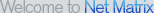Linear Algebra 2

Performs computations associated with matrices, including solution of linear systems of equations (even least squares solution of over-determined or inconsistent systems and solution by LU factors), matrix operations (add, subtract, multiply), finding the determinant, trace, inverse, adjoint, QR or LU factors, eigenvalues and eigenvectors, establish the definiteness of a symmetric matrix, perform scalar multiplication, transposition, shift, create matrices of zeroes or ones, identity, symmetric, general, random matrices, etc.

• Linear Algebra Screenshot